# Arithmetic and Logical Operations of 8086 Microprocessor

In this article, we are going to discuss in brief about the arithmetic and logical instruction/ operations of 8086 microprocessor.
Submitted by Uma Dasgupta, on March 22, 2019

## Logical Instructions

a) AND: Logical AND

Atleast one of the operant should be a register or a memory operant both the operant cannot be a memory location or immediate operant.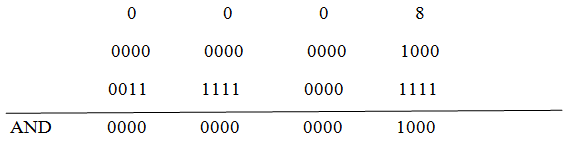b) OR: logical OR

Both inputs are low in this case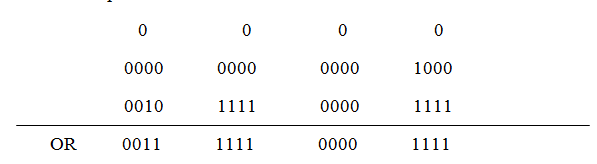c) NOT: logical invert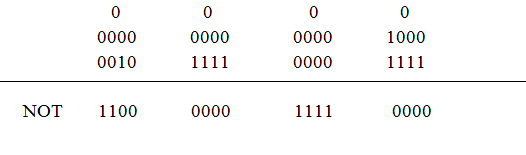d) XOR: logical Exclusive OR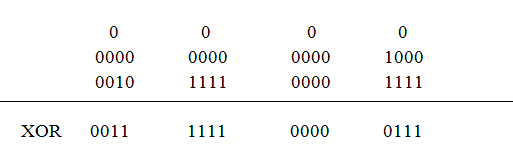e) TEST instruction: Logical compare instruction

It will do bit by bit AND operation on the operands. Each bit of the result is then said to be 1 if the corresponding bit else the result bit is said to zero.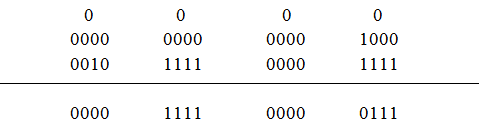f) SHL: Shift left logical

It will shift the operant bit by bit to the left and insert the zero in the newly introduced least significant bits. Here operant is not an immediate data.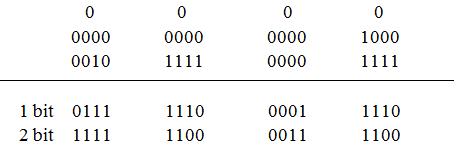g) SHR: Shift right logical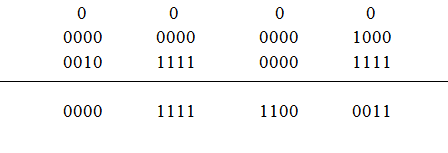h) SAR: Shift Right Arithmetic

Same as the logical shift but it inserts the most significant bit of the operand in the newly inserted position.

i) ROR (Rotate Right without carry)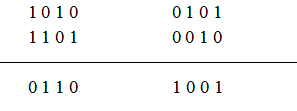j) ROL (Rotate left without carry)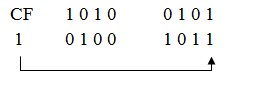k) RCR ( Rotate right through carry)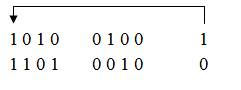l) RCL (Rotate left through carry)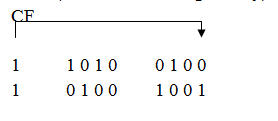## Arithmetic Operations

In this operation we perform sum of data in two different registers.

Memory to memory operation is not possible. Source and destination operant cannot be a memory location.

Also the content of segment register cannot be added.

(3) INC ( Increment)

Increment the data by 1 and immediate data cannot be an operand here.

(4) DEC ( decrement by 1)

Decreases data by 1 and immediate data cannot be an operand.

(5) SUB

It will subtract source operand from destination and result is stored in destination.

Both operands may not be a memory operand and destination operand must not be an immediate data.

(6) SBB (Subtract with borrow)

Borrow flag/ Carry flag is also subtracted.

(7) CMP (compare)

For comparison it subtract source operand from the destination, but result is not stored anywhere.

(8) ASS (ASCII adjust AL after subtraction)

It converts the result of subtraction of two valid unpacked BCD digits to a single valid BCD no. and takes AL as implicit operand.

(9) AAM (ASCII adjust after multiplication)

AAM converts the result of multiplication of two valid BCD digits to a single valid BCD no. takes AC as implicit operand.

It unpacks the result by dividing AX by 10 placing the quotient into AH and remainder in AL.

It converts unpacked BCD digits in AH and AL register in a single binary number in AX register in preparation for a division operation.

Before executing AAD placed most significant BCD digit in the AH register and least significant digit in AL register. Then the two BCD digits are combined in a single binary number by setting.

Used to convert the result of the addition of 2 packed BCD no. to valid BCD no. if lower nibble AL > 9.

(12) DAS (Decimal address after subtraction)

It converts the result of subtraction of two packed number to a valid BCD no.

Conclusion:

In this article I have discussed briefly about almost all arithmetic and logical instructions of 8086 microprocessor. I hope they have covered all we need to know about the operations performed by 8086 microprocessor if you have any further queries shoot them in the comment section below. See you in my next article till then stay healthy and keep learning!